Courses

# Z-Transform - 1

## 10 Questions MCQ Test Topicwise Question Bank for Electronics Engineering | Z-Transform - 1

Description
This mock test of Z-Transform - 1 for Electrical Engineering (EE) helps you for every Electrical Engineering (EE) entrance exam. This contains 10 Multiple Choice Questions for Electrical Engineering (EE) Z-Transform - 1 (mcq) to study with solutions a complete question bank. The solved questions answers in this Z-Transform - 1 quiz give you a good mix of easy questions and tough questions. Electrical Engineering (EE) students definitely take this Z-Transform - 1 exercise for a better result in the exam. You can find other Z-Transform - 1 extra questions, long questions & short questions for Electrical Engineering (EE) on EduRev as well by searching above.
QUESTION: 1

Solution:
QUESTION: 2

Solution:
QUESTION: 3

### The z-transform of a signal is given by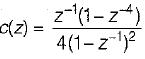Its final valve is

Solution:
QUESTION: 4

Which one of the following represents the impulse response of a system defined by H(z) = z-m

Solution:
QUESTION: 5

The z-transform of the time function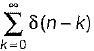is

Solution:
QUESTION: 6

The z-transform of the system is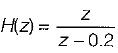if the ROC is |z| < 0.2, then the impulse response of the system is

Solution:
QUESTION: 7

If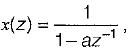the two discrete time signal

Solution:
QUESTION: 8

lf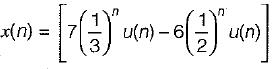The ROC for the above discrete time signal is

Solution:
QUESTION: 9

A stable and causal system is described by the difference equation,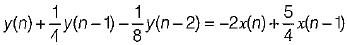The impulses response of the system is

Solution:
QUESTION: 10

A linear discrete time system has the characteristics equation, z3 - 0.81 z = 0, the system is

Solution: